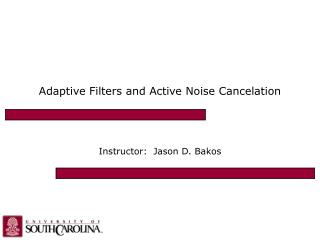DownloadDownload PresentationAdaptive Filters and Active Noise Cancelation

Adaptive Filters and Active Noise Cancelation

Télécharger la présentationAdaptive Filters and Active Noise Cancelation

- - - - - - - - - - - - - - - - - - - - - - - - - - - E N D - - - - - - - - - - - - - - - - - - - - - - - - - - -
Presentation Transcript

1. Adaptive Filters and Active Noise Cancelation Instructor: Jason D. Bakos

2. Signals • Represented as a mapping between time and magnitude Dt freq=1/Dt • Sample at regular intervals .5Dt sample rate=2/Dt

3. Signals • Sampling allows a signal to be represented as a discrete function over non-negative integers: • s(n) • vector form [0.243 1.231 0.445 3.122] aliasing adding harmonics of the sampling frequency to a sampled signal makes it appear the same

4. The FIR Filter • Finite Impulse Response • Set of coefficients that change a signal through convolution • M-1 is the order (memory) of the filter • Example • b = [.2 .1 .5] • x = [1 5 2 4 3] • y= [.2 1.1 1.4 3.5 2.0] • Zero-pad the right side of the signal value and we do convolution • Example: (2x2 + 4x + 3) * (4x2 + 3x + 2)

5. The FIR Filter • Filter the unit impulse reponse [1 0 0 0 …] • Result is the coefficients • Assume: • bn = 1/M for all n • Averaging filter M=8 M=16 M=24

6. Averaging Filter on Sinusoids M=8 M=16 M=24

7. x(5) x(4) x(3) x(2) x(1) x(4) x(3) x(2) x(1) x(2) x(1) x(3) x(2) x(1) x(6) x(5) x(4) x(3) x(2) reg reg reg reg reg b1 b2 b3 b4 b5 18 18 X X X X X 36 48 48 out + + + + DSP48 FIR Implementation • Single-cycle (parallel) implementation: x(1)

8. = 0 reg FIR Implementation • Multi-cycle (and software) implementation: 0 to M counter rst x addressable FIFO out X + coefficients

9. LMS Adaptive Filtering GOAL: Set the coefficients of F(x) to minimize e(x) d(n) x(n) S + - x(n) y(n) GOAL: Set the coefficients to minimize e(n) FIR e(n) LMS m can be fixed or normalized:

10. MATLAB Example of LMS ANC • Load some speech and generate noise % load the signal signal = load('RFrost.txt'); % play it myplayer = audioplayer(signal,10000); playblocking(myplayer); % create noise % % white noise %gain = .5; %noise = gain * randn(size(signal,1),1); % % sinusoid spacing F0 = 60; A = [0.01 0.01 0.02 0.2 0.3 0.4 0.3 0.2 0.1 0.07 0.02 0.01]; noise = zeros(length(signal),1); for k = 1:length(A) noise = noise + (A(k)*sin(2*pi*F0*k/10000*(1:length(signal))+rand(1)))'; end % create the "sound" sound = signal + noise; % play the sound myplayer = audioplayer(sound,10000); playblocking(myplayer);

11. MATLAB Example of LMS ANC • Initialize the noise filter % set the filter parameters and initialize the filter mu = .2; taps = 100; coef = zeros(1,taps); % pad the noise noise = [zeros(taps-1,1);noise]; error_out = []; out_signal = []; • Train the noise filter i = taps; while i <= size(noise,1) % compute filter output out = fliplr(coef)*noise(i-taps+1:i,1); out_signal = [out_signal;out]; % compute the error and record it error = sound(i-taps+1,1) - out; error_out = [error_out;error]; % update the step size and coefficients with nLMS step_size = mu / (noise(i-taps+1:i,1)' * noise(i-taps+1:i,1)); %step_size = mu; coef = coef + step_size * fliplr(noise(i-taps+1:i,1)') * error; i = i + 1; end

12. MATLAB Example of LMS ANC • Play and plot % play and plot the errror myplayer = audioplayer(error_out,10000); playblocking(myplayer); n=1:length(signal); plot(n,sound',n,out_signal',n,error_out'); legend('signal + error','anti-noise','result');

13. Filtered-X LMS ANC x(n) d(n) S + - x(n) y’(n) y(n) actual secondary path filter FIR est. secondary path filter e(n) x’(n) LMS

14. MATLAB Example of Filtered-X ANC • Create the secondary path response % create the secondary path response % delay is 7 samples delayS = 10; N = 100; % order=9, 200 Hz to 2500 Hz, atten. in stop bands=20 [b,a] = cheby2(9,20,[200 2500]/5000); % extract impulse response impulse = [zeros(1,delayS) log(0.99*rand(1,N-delayS)+0.01) .* sign(randn(1,N-delayS)) .* exp(-0.01*(1:N-delayS))]; H = filter(b,a,impulse); H = H/norm(H); • Listen to the secondary path response % play the sound myplayer = audioplayer(sound,10000); playblocking(myplayer); % create and play the sound received from the error mic received_sound = filter(H,1,sound); myplayer = audioplayer(received_sound,10000); playblocking(myplayer);

15. MATLAB Example of Filtered-X ANC • “Learn” the secondary path response % learn the secondary path filter and filter the received noise white_noise = randn(1,30000); received_noise = filter(H,1,white_noise); h=adaptfilt.nlms(250,.1,1,.1); [yS,eS] = filter(h,white_noise,received_noise); • Filter X! ref. error % filter reference with 'our' filter % this is what implements filtered-x noise_filtered=filter(h.coefficients,1,noise); • Initialize the noise filter % set the filter parameters and initialize the filter mu = .0001; taps = 350; coef = zeros(1,taps); % pad the noise noise = [zeros(taps-1,1);noise]; noise_filtered = [zeros(taps-1,1);noise_filtered]; error_out = []; error_filtered_out = []; out_signal = zeros(length(H)-1,1);

16. MATLAB Example of Filtered-X ANC • Train the noise filter i = taps; j = length(H); while i <= size(noise,1) % compute filter output out = fliplr(coef)*noise(i-taps+1:i,1); out_signal = [out_signal;out]; % filter the filter output out_filtered = fliplr(H) * out_signal(j-length(H)+1:j,1); j = j + 1; % compute the error and record it error = sound(i-taps+1,1) - out_filtered; error_out = [error_out;error]; % update the step size and coefficients with nLMS %step_size = mu / (noise_filtered(i-taps+1:i,1)' * noise_filtered(i-taps+1:i,1)); step_size = mu; coef = coef + step_size * fliplr(noise_filtered(i-taps+1:i,1)') * error; i = i + 1; % debug if mod(i,2000) == 0 coef end end

17. MATLAB Example of Filtered-X ANC